# MESH (Command)

Creates a 3D mesh primitive object such as a box, cone, cylinder, pyramid, sphere, wedge, or torus.

Find

The basic mesh forms, known as mesh primitives, are the equivalent of the primitive forms for 3D solids.

You can reshape mesh objects by smoothing, creasing, refining, and splitting faces. You can also drag edges, faces, and vertices to mold the overall form.

Note: By default, new mesh primitives are created with no smoothness. To change the default smoothness, enter MESH at the Command prompt. Then specify the Settings option before you specify the type of mesh primitive you want to create.

The following prompts are displayed.

### Box

Creates a 3D mesh box.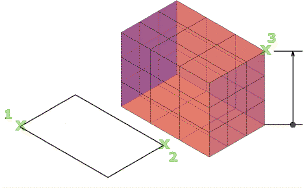Specify the length of the sides.

First corner / Corner

Sets one corner of the mesh box.

Center

Sets the center of the mesh box.

Cube

Sets all edges of the box to be of equal length.

Length

Sets the length of the mesh box along the X axis.

Width

Sets the width of the mesh box along the Y axis.

Height

Sets the height of the mesh box along the Z axis.

2Point (height)

Sets the height based on the distance between two points.

### Cone

Creates a 3D mesh with a circular or elliptical base that tapers symmetrically to a point or to a planar face.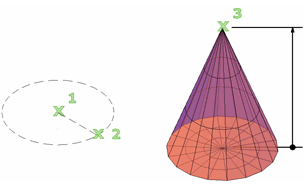Specify the diameter and height.

Center point of base

Sets the center point of the base of the mesh cone.

3P (three points)

Sets the location, size, and plane of the mesh cone by specifying three points. The third point sets the size and planar rotation of the cone base.

2P (diameter)

Defines the base diameter of the mesh cone based on two points.

Defines the base of the mesh cone with a specified radius that is tangent to two points on objects:

• Point on object for first tangent. Sets a point on an object to serve as the first tangent point.
• Point on object for second tangent. Sets a point on an object to serve as the second tangent point.
• Radius of circle. Sets the radius of the mesh cone base.

If the specified criteria can produce multiple results, the closest tangent points are used.

Elliptical

Specifies an elliptical base for the mesh cone.

• Endpoint of first axis. Sets the start point for the first axis of the mesh cone base and then specifies the other axis endpoints.
• Center. Specifies the method for creating an elliptical mesh cone base that starts with the center point of the base. You then set the distance to the first axis (the radius) and the endpoint of the second axis.

Sets the radius of the mesh cone base.

Diameter

Sets the diameter for the base of the cone.

Height

Sets the height of the mesh cone along an axis that is perpendicular to the plane of the base.

2Point (height)

Defines the height of the mesh cone by specifying the distance between two points.

Axis endpoint

Sets the location of the top point of the cone or the center of the top face of a cone frustum. The orientation of the axis endpoint can be anywhere in 3D space.

Specifies the top radius of the cone, creating a cone frustum.

### Cylinder

Creates a 3D mesh cylinder.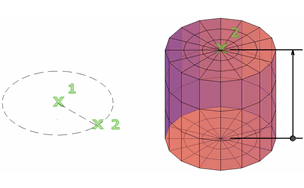Specify the size of the base and height.

Center point of base

Sets the center point of the mesh cylinder base.

3P (three points)

Sets the location, size, and plane of the mesh cylinder by specifying three points. The third point sets the size and planar rotation of the mesh cylinder base.

2P (diameter)

Sets the diameter of the mesh cylinder base by specifying two points.

2Point (height)

Defines the height of the mesh cylinder by specifying the distance between two points.

Defines the base of the mesh cylinder with a specified radius that is tangent to two objects. If the specified criteria can produce multiple results, the closest tangent points are used.

Sets the radius of the mesh cylinder base.

Diameter

Sets the diameter for the base of the cylinder.

Height

Sets the height of the mesh cylinder along an axis that is perpendicular to the plane of the base.

Axis endpoint

Sets the location of the top face of the cylinder. The orientation of the axis endpoint can be anywhere in 3D space.

Elliptical

Specifies an elliptical base for the mesh cylinder.

• Endpoint of first axis. Sets the start point and endpoint for the first axis of the mesh cone base. Then sets the height (second axis endpoint).
• Center. Specifies the method for creating an elliptical mesh cone base that starts with the center point of the base and specifies the radius and the height.

### Pyramid

Creates a 3D mesh pyramid.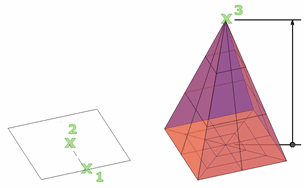Specify the diameter and height.

Center point of base

Sets the center point of the mesh pyramid base.

Edge

Sets the length of the one edge of the mesh pyramid base, as indicated by two points that you specify.

Sides

Sets the number of sides for the mesh pyramid. Enter a positive value from 3-32.

Sets the radius of the mesh pyramid base.

Inscribed

Specifies that the base of the mesh pyramid is inscribed, or drawn within, the base radius.

Height

Sets the height of the mesh pyramid along an axis that is perpendicular to the plane of the base.

2Point (height)

Defines the height of the mesh cylinder by specifying the distance between two points.

Axis endpoint

Sets the location of the top point of the pyramid or the center of the top face of a pyramid frustum. The orientation of the axis endpoint can be anywhere in 3D space.

Specifies the top radius of the mesh pyramid, creating a pyramid frustum.

Circumscribed

Specifies that the base of the pyramid is circumscribed, or is drawn around, the base radius.

### Sphere

Creates a 3D mesh sphere.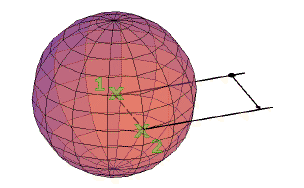Specify the size of the sphere (diameter or radius).

Center point

Sets the center point of the sphere.

• Diameter. Creates a mesh sphere based on a specified diameter.
3P (three points)

Sets the location, size, and plane of the mesh sphere by specifying three points.

2P (diameter)

Sets the diameter of the mesh sphere by specifying two points.

Defines a mesh sphere with a specified radius that is tangent to two points on objects:

If the specified criteria can produce multiple results, the closest tangent points are used.

### Wedge

Creates a 3D mesh wedge.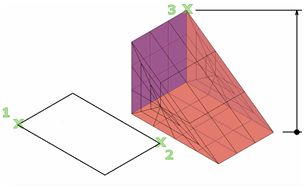Specify the length and width of the base and the height.

First corner

Sets the first corner of the mesh wedge base.

Other corner
Sets the opposite corner of the mesh wedge base, located on the X,Y plane.
Center

Sets the center point of the mesh wedge base.

Cube

Sets all edges of the mesh wedge base to be of equal length.

Length

Sets the length of the mesh wedge base along the X axis.

Width

Sets the width of the mesh box along the Y axis.

Height

Sets the height of the mesh wedge. Enter a positive value to draw the height along the positive Z axis of the current UCS. Enter a negative value to draw the height along the negative Z axis.

2Point (height)

Defines the height of the mesh wedge by specifying the distance between two points.

### Torus

Creates a 3D mesh primitive torus.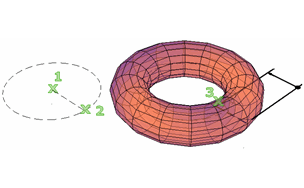Specify two values: the size of the tube and the distance from the center of the torus to the center of the tube.

Center point

Sets the center point of the mesh torus.

3P (three points)

Sets the location, size, and rotation of the mesh torus by specifying three points along the path that the tube passes through.

2P (torus diameter)

Sets the diameter of the mesh torus by specifying two points. The diameter is calculated from the center point of the torus to the center point of the tube.

Defines a mesh torus radius that is tangent to two objects. The specified tangent points are projected into the current UCS.

If the specified criteria can produce multiple results, the closest tangent points are used.

Sets the radius of the mesh torus, measured from the center point of the torus to the center point of the tube.

Diameter (torus)

Sets the diameter of the mesh torus, measured from the center point of the torus to the center point of the tube.

Sets the radius of the profile that is swept around the mesh torus path.

Sets the radius of the profile of the tube based on the distance between two points.

Tube diameter

Sets the diameter of the profile of the mesh torus tube.

### Settings

Modifies the smoothness and tessellation values for the new mesh object.

Level of smoothness

Sets the initial degree of smoothness, or roundness, to be applied to the mesh. Enter 0 to eliminate smoothness. Enter a positive integer up to 4 for increased degrees of smoothness. The value is retained for the current drawing session.

This smoothness value is limited by the value of SMOOTHMESHMAXLEV.

Tessellation

Opens the Mesh Primitive Options dialog box, where you can update tessellation values (the number of faces) for each dimension of a mesh primitive option.